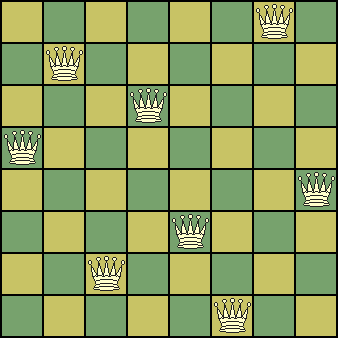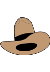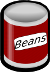#Answers

Math Lair Home > Puzzles & Problems > Answers

Here are answers to various questions posed on various other pages:

Other answer pages: Baseball Cards Answers | Lateral thinking puzzle answers, page 1 | page 2 | page 3 | page 4 | page 5 | One-Dollar Words Answers | Pigeonhole Principle Problems Answers | Rockets Answers
Alphametics
1. 29786 + 850 + 850 = 31486.
2. 526485 + 197485 = 723970.
3. 9762 + 2617 = 12379.
4. 21978 × 4 = 89712.
5. 345 × 986 = 340170, or 218 × 965 = 210370, or 165 × 972 = 160380.
6. 7641 − 1467 = 6174
The Digital Clock
48 minutes, from 12:12:59.9999... to 1:01:00. While the time from 4:04 to 5:05 is 61 minutes, we can find a shorter time, between 12:12 and 1:01. The time between 12:12 and 1:01 is 49 minutes, but we need to subtract 1 minute since 12:12 will be displayed for a full minute.
The Disadvantages of Being Absent-minded
The professor had completely forgotten that two of the other students, who always sat next to each other, were identical twins.
Don't Make a Triangle
1. The game would take 15 turns.
2. There are 6C3 = 20 possible triangles that could be drawn.
3. One player will always win.
4. The proof is by contradiction. Assume that the game is tied and that all possible lines have been drawn and no triangle exists. Now, pick any one dot. It will have five lines drawn from it. Since there are five lines and two colours, by the pigeonhole principle at least three of those lines must be the same colour. Now, consider the three points connected with lines of that colour. Now it must be the case that either (a) one pair of those points is connected with a line of the same colour, in which case a triangle is made using the original point and that pair of points, or (b) all of those points are connected with lines of a different colour, in which case a triangle of that colour is made between them. Either way, a triangle exists, which contradicts our original assumption that no triangle exists. So, our initial assumption must be false and the game cannot end in a draw.
5. This problem is completely analogous to the previous problem if you take each of the people to be a dot, two people being acquainted being a line of one colour and two people being strangers being a line of another colour. Just as a tringle of one colour or another must exist, either three people are mutually acquainted or three people are mutual strangers.
6. As this is a combinatorial game, and it cannot be drawn, then one player must have a winning strategy.
Eight Queens Puzzle
One of the 12 unique solutions is shown below:Einstein's Riddle
The Norwegian drinks water. The Japanese owns the zebra.
Encircling the Earth
5.76". Surprising, eh? Think about it.
Hole In One
Based on the information in the problem, and assuming that the odds of making a hole in one on any one hole are independent of the odds of doing so on another hole, the odds of getting two holes in one in a round of golf (18 holes) are
(182) × (112,500)²
= 9.792 × 10−7, or around one in a million.
How Many Days 'Til Christmas?
November 12. In order to display days 0 through 9, all 10 digits have to be on one cube or another; however, since "6" looks like "9" upside-down, we can save a digit by making "6" do double duty. This leaves us with three spare digits on one cube or another. In order to represent the numbers 11, 22, and 33, we will need to put 1, 2, and 3 on both cubes. So, one cube could have the digits 0, 1, 2, 3, 5, and 6, and the other the digits 1, 2, 3, 4, 7, and 8 (we have to put 0 and 4 on different cubes in order to represent 40, but 5, 6, 7, and 8 can be on either cube). This allows us to represent all numbers between 0 and 43. 43 days before Christmas is November 12, so we can start using the decoration on that day.
How Much for a Turkey?
Assume that each of the 72 turkeys cost the exact same (i.e. the turkeys weren't sold by weight) and that price is an integer number of cents. Therefore, _679_ must be divisible by 72. If a number is divisible by 72 it must be divisible by both 8 and 9. Using the tests for divisibility, a number is divisible by 8 only if one of the two hold:
1. The hundreds digit is even and the last two digits are a multiple of 8.
2. The hundreds digit is odd and the last two digits are a multiple of 4 but not 8.

Since the hundreds digit is odd, the last two digits must be a multiple of 4 but not 8. The only number to put in the second blank to make this true would be 2. We now have _6792.

Now, the number _6792 must be divisible by 9, so its digits must add to a multiple of 9. To accomplish this, the first blank must be 3. Therefore, the 72 turkeys cost \$367.92 and each turkey cost \$5.11.

The Hymn Board
I'm not sure what the best answer is; the best answer that I came up with is \$73.50.
• To start with, the church will need at least 11 copies of the numbers 1 through 7 (since, using 7 as an example, hymns 177, 277, 377, 477, and 777 could be selected) and 10 copies of the numbers 8, 9, and 0. So far, we are at 107 plates.
• One savings that could be made is to note that "6" looks like "9" upside-down, so instead of ordering 11 copies of 6 and 10 copies of 9, it will be sufficient to order 14 copies of 6 (14 copies are required because hymns 666, 669, 696, 699, and one containing two copies of 6 or 9 could be used). This brings us to a total of 100 plates.
• Another savings is, instead of printing 10 copies of "0", it is sufficient to print 5 copies of "0" and 3 copies of "00" (since the hymn number never starts with 0). Similarly, instead of printing 10 copies of "8", print 5 copies of "8" and 3 copies of "88". So far, we are at 102 digits on 96 plates.
• Finally, digits can be printed on both sides of the plates, saving half of the required number of plates. The church will still be able to represent all combinations as long as the same number is not printed on both sides of a plate, and as long as there is a variety of numbers on the opposite side of each number (e.g. not all plates with "7" on one side have, say, "5" on the opposite side, etc.). With these savings, 102 digits will be printed on 48 plates, for a cost of 102 × \$0.25 + 48 × \$1 = \$73.50.
Metapuzzles
1. If the second twin answered "Yes," then one of them would be telling the truth and one would be lying, but we couldn't tell which. So, the second twin answered "No." Now, if John was the first twin, they would both have been telling the truth, but the question states that one always lies. So, they both lied and the second twin is John.
2. This problem can be solved by working backwards, by assuming each is the murderer and seeing how many statements are true:
• If Abby is the killer, 3 statements are true.
• If Bernard is the killer, 1 statement is true.
• If Claire is the killer, 2 statements are true.
• If Damian is the killer, 2 statements are true.
• If Edward is the killer, 3 statements are true.
• If someone else is the killer, 2 statements are true.
If the police officer were told that 2 statements were true, he would not be able to distinguish between Claire, Damian, or someone else. If he were told that 3 statements were true, he would not be able to distinguish between Abby and Edward. The only way in which he could determine the murderer is if he were told that 1 statement was true, in which case Bernard is the murderer.
3. This problem can be solved by going through each possibility. If the logician had been told that A said that C was the liar, there are nine possibilities depending on what B and C said. In all nine possibilities, either B is the only one who could be convicted or there are multiple possibilites so the judge wouldn't have been able to tell. However, we know that the judge could tell, so the logician would know that the judge convicted B if he were told that A said that C was the liar. Now, if the logician had been told that A said that C was the spy, then the spy could have been A (if, say, B said that A was a truth-teller and C said that B was a liar), or it could have been B (if, say, B said that A was a truth-teller and C said that B was the spy). So, the logician couldn't figure out who the judge convicted if he were told that A said that C was the spy. So, he must have been told that C was the liar, and so B is the spy.
Miscellaneous puzzles
1. Exactly one of the politicians is honest.
2. The only one whose stamp can be deduced is C. Because A answered "no," we know that B and C do not have both Red or Yellow stamps. So, if B saw C with either Red or Yellow, he would know that he does not have the same colour. Therefore, C must have a Green stamp.
3. \$0.50.
4. \$5.
Miscellaneous puzzles 2
1. 27. The easiest way to solve this problem is to consider that each player, except for the winner of the tournament, will lose exactly once. Since each match has one loser, there must be 27 matches.
2. The amount of wine in the water bottle is the same as the amount of water in the wine bottle. Each bottle currently contains a litre of liquid. So, (amount of water in the water bottle) + (amount of wine in the water bottle) = 1 litre. Since none of the original wine has been lost, (amount of wine in the wine bottle) + (amount of wine in the water bottle) = 1 litre. So, the amount of water in the water bottle equals the amount of wine in the wine bottle, and so the amount of wine in the water bottle equals the amount of water in the wine bottle.
3. \$1.38. Surprising, eh?
Phone Equations
These were my answers; you may have found another equation. If so, good job!
1. −5 + 7 − 6 = 54 − 58
2. 3 ÷ 27 = 6 ÷ (9 × (5 + 1))
3. Answers may vary.
Riddles
1. A quarter and a nickel. One of them is not a nickel, and the other one is.
2. All of them have 28 days.
3. Neither. Seven and five are twelve.
4. You would light the match first.
5. If you take three apples from five apples, you have three apples.
6. If you put them all together, you have one big haystack.
7. Only once; after that, the number is no longer 170.
8. There are twelve four-cent stamps in a dozen.
9. Nine were left.
10. None; when you dig a hole, you remove the dirt.